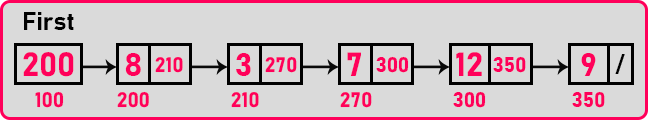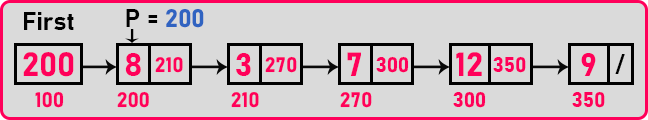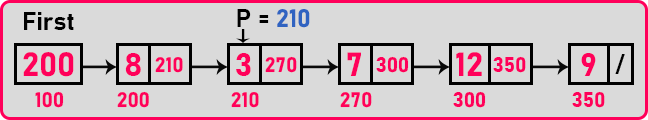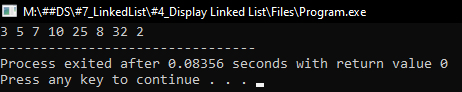# How to Display a Linked List in C

## How to Display a Linked List in C Language?

In this article, I am going to discuss How to Display a Linked List in C Language with Examples. Please read our previous article, where we discussed What is Linked List Data Structure with Examples.

##### How to Display a Linked List in C Language:

If we have ordered a linked list then how to display all the elements? For this, we should know how to traverse a linked list. Traversing means visiting all the nodes of a linked list. So let us see how to traverse.For visiting all the nodes one by one, we must start from 1st node. So, this pointer ‘First’ will give the address of 1st node we should not modify first. We should take one more temporary pointer and we should take the help of that pointer. So let us take ‘p’ pointing on this 1st node.struct Node *p = first;

With this line of code, ‘p’ will be a pointer pointing on the same node where the first is pointing. So, we’re on the 1st node, let us move to the next node. How to move ‘p’ to the next node?

p = p.next;

It will move ‘p’ to the next node. We have already seen this statement in the previous article.We can again go to the next node by writing ‘p = p.next’ and in this way, we will access the next node in a linked list. It should be done repeatedly in a loop to traverse the whole linked list. So, we will use a while loop. Till where do we want to repeat the statement? At last node. So, at the last node, we will stop to move further. We can write the condition as,

while (p != 0){
p = p.next;
}

Here we have mention the condition ‘p != 0’ or ‘p != NULL’ not for termination but continuation. Termination is when ‘p’ is equal to zero. We should continue as long as ‘p’ is not equal to zero.

##### Why we are using the while loop, not for loop?

Because we don’t know how many nodes are there. If we know how many times, we have to repeat then we can use for loop also.

This is loop is for traversing the nodes of a linked list. So, while traversing we can do a lot of things. For example – displaying the nodes, counting the nodes, adding all the elements, searching for elements, sorting the elements, and more other operations. So, these operations we will see in upcoming articles. Here we are just showing you display the elements of the linked list.

while (p != 0){
printf(“%d”, p->data);
p = p.next;
}

This will print the whole linked lists. So, this is the code for displaying a linked list. We can even make it as a function,

Display (struct Node *p){
while (p != 0){
printf(“%d”, p->data);
p = p.next;
}
}

You can call this function bypassing the first pointer. So, this is for displaying the linked list. Let us see the full code for displaying a linked list in C language.

##### Program to Display Linked List in C Language:
```#include <stdio.h>
#include <stdlib.h>
struct Node
{
int data;
struct Node *next;
}   *first = NULL;

void create(int A[], int n)
{
int i;
struct Node *t, *last;
first = (struct Node *) malloc(sizeof(struct Node));
first->data = A;
first->next = NULL;
last = first;

for (i = 1; i < n; i++)
{
t = (struct Node *) malloc(sizeof(struct Node));
t->data = A[i];
t->next = NULL;
last->next = t;
last = t;
}
}

void Display(struct Node *p)
{
while (p != NULL)
{
printf ("%d ", p->data);
p = p->next;
}
}

int main()
{
struct Node *temp;
int A[] = { 3, 5, 7, 10, 25, 8, 32, 2 };
create(A, 8);
Display (first);
return 0;
}
```
###### Output:In the next article, I am going to discuss the Recursive Function for Displaying a Linked List in C Language with Examples. Here, in this article, I try to explain How to Display Linked List in C Language with Examples and I hope you enjoy this How to Display Linked List in C Language with Examples article.